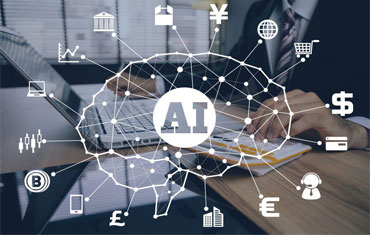### Artificial Intelligence Training In OMR – Sholinganallur – ChennaiAutomation Minds is a leading provider of Artificial Intelligence (AI) Training in Chennai. India’s Top Rated AI Training Institute offers realtime practical Artificial Intelligence Training with realtime project, job orientation and certification guidance. Our trainings are 100% job assured.

Awarded as the Best Artificial Intelligence and Machine Learning Training Center in Chennai – We Guarantee Your AI Training Success in Chennai

At Automation Minds, selected people get trained by industry experts who work on Artificial Intelligence and Machine Learning techniques every single day.

Introduction to Deep Learning

Learning Objectives:

In this module, you’ll get an introduction to Deep Learning and understand how Deep Learning solves problems which Machine Learning cannot. Understand the fundamentals of Machine Learning and relevant topics of Linear Algebra and Statistics.

Topics:

Deep Learning: A revolution in Artificial Intelligence

Limitations of Machine Learning

What is Deep Learning?

The advantage of Deep Learning over Machine learning

3 Reasons to go for Deep Learning

Real-Life use cases of Deep Learning

Review of Machine Learning: Regression, Classification, Clustering, Reinforcement Learning, Underfitting and Overfitting, Optimization

Hands-On
Implementing a Linear Regression model for predicting house prices from Boston dataset

Implementing a Logistic Regression model for classifying Customers based on an Automobile purchase dataset.

Understanding Neural Networks with TensorFlow.

Learning Objectives:

In this module, you’ll get an introduction to Neural Networks and understand it’s working i.e. how it is trained, what are the various parameters considered for its training and the activation functions that are applied.

Topics:

How Deep Learning Works?

Activation Functions

Illustrate Perceptron

Training a Perceptron

Important Parameters of Perceptron

What is TensorFlow?

TensorFlow code-basics

Graph Visualization

Constants, Placeholders, Variables

Creating a Model

Step by Step – Use-Case Implementation

Hands-On

Building a single perceptron for classification on SONAR dataset

Deep dive into Neural Networks with TensorFlow

Learning Objectives:

In this module, you’ll understand the backpropagation algorithm which is used for training Deep Networks. You will know how Deep Learning uses neural network and backpropagation to solve the problems that Machine Learning cannot.

Topics:

Understand the limitations of a Single Perceptron

Understand Neural Networks in Detail

Illustrate Multi-Layer Perceptron

Backpropagation – Learning Algorithm

Understand Backpropagation – Using Neural Network Example

MLP Digit-Classifier using TensorFlow

TensorBoard

Hands-On

Building a multi-layered perceptron for classification of Hand-written digits

Master Deep Networks

Learning Objectives:

In this module, you’ll get started with the TensorFlow framework. You will understand how it works, its various data types & functionalities. In addition, you will create an image classification model.

Topics:

Why Deep Networks

Why Deep Networks give better accuracy?

Use-Case Implementation on SONAR dataset

Understand How Deep Network Works?

How Backpropagation Works?

Illustrate Forward pass, Backward pass

Types of Deep Networks

Hands-On

Building a multi-layered perceptron for classification on SONAR dataset

Convolutional Neural Networks (CNN)

Learning Objectives:

In this module, you’ll understand convolutional neural networks and its applications. You will learn the working of CNN, and create a CNN model to solve a problem.

Topics:

Introduction to CNN’s

CNN’s Application

The architecture of a CNN

Convolution and Pooling layers in a CNN

Understanding and Visualizing a CNN

Hands-On
Building a convolutional neural network for image classification. The model should predict the difference between 10 categories of images.

Recurrent Neural Networks (RNN)

Learning Objectives:

In this module, you’ll understand Recurrent Neural Networks and its applications. You will understand the working of RNN, how LSTM are used in RNN, what is Recursive Neural Tensor Network Theory, and finally, you will learn to create an RNN model.

Topics:

Introduction to RNN Model

Application use cases of RNN

Modelling sequences

Training RNNs with Backpropagation

Long Short-Term Memory (LSTM)

Recursive Neural Tensor Network Theory

Recurrent Neural Network Model

Hands-On

Building a recurrent neural network for SPAM prediction.

Restricted Boltzmann Machine (RBM) and Autoencoders

Learning Objectives:

In this module, you’ll understand RBM & Autoencoders along with their applications. You will understand the working of RBM & Autoencoders, illustrate Collaborative Filtering using RBM and understand what are Deep Belief Networks.

Topics:

Restricted Boltzmann Machine

Applications of RBM

Collaborative Filtering with RBM

Introduction to Autoencoders

Autoencoders applications

Understanding Autoencoders

Hands-On

Building an Autoencoder model for classification of handwritten images extracted from the MNIST Dataset, Keras API

Learning Objectives:

In this module, you’ll understand how to use Keras API for implementing Neural Networks. The goal is to understand various functions and features that Keras provides to make the task of neural network implementation easy.

Topics:

Define Keras

How to compose Models in Keras

Sequential Composition

Functional Composition

Predefined Neural Network Layers

What is Batch Normalization

Customizing the Training Process

Using TensorBoard with Keras

Use-Case Implementation with Keras

Hands-On

Build a model using Keras to do sentiment analysis on twitter data reactions on GOP debate in Ohio, TFLearn API

Learning Objectives:

In this module, you’ll understand how to use TFLearn API for implementing Neural Networks. The goal is to understand various functions and features that TFLearn provides to make the task of neural network implementation easy.

Topics:

Define TFLearn

Composing Models in TFLearn

Sequential Composition

Functional Composition

Predefined Neural Network Layers

What is Batch Normalization

Customizing the Training Process

Using TensorBoard with TFLearn

Use-Case Implementation with TFLearn

Hands-On

Build a recurrent neural network using TFLearn to do image classification on hand-written digits

In-Class Project

Learning Objectives:

In this module, you should learn how to approach and implement a  project end to end. The instructor will share his industry experience and related insights that will help you kickstart your career in this domain. In addition, we will be having a QA and doubt clearing session for you.

Topics:

How to approach a project?

Hands-On project implementation

What Industry expects?

Industry insights for the Machine Learning domain

QA and Doubt Clearing Session